# How to know the number of the week?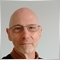802

Hello,

How to know the number of the week?

MqlDateTime gives a lot of info, but I did not find the number of the week.

```//+------------------------------------------------------------------+
//|                                                   WeekNumber.mq5 |
//|                                   Copyright 2018, Pierre Rougier |
//|                                        http://apprendre-mql5.com |
//+------------------------------------------------------------------+
#property copyright "Copyright 2018, Pierre Rougier"
#property link      "http://apprendre-mql5.com"
#property version   "1.00"
//+------------------------------------------------------------------+
//| Script program start function                                    |
//+------------------------------------------------------------------+
void OnStart()
{
//---

MqlDateTime dow;
TimeCurrent(dow);

Alert("dow.year :"+dow.year);
Alert("dow.mon :"+dow.mon);
Alert("dow.day :"+dow.day);
Alert("day_of_week :"+dow.day_of_week);
Alert("dow.day_of_year :"+dow.day_of_year);

}
//+------------------------------------------------------------------+
```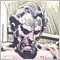5418

Pierre Rougier:

Hello,

How to know the number of the week?

MqlDateTime gives a lot of info, but I did not find the number of the week.

https://www.mql5.com/en/articles/599#week_number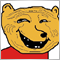1086

`int(1+dow.day_of_year/7)`5418

kypa:
`int(1+dow.day_of_year/7)`

Well, consider January 4, 2016.

The fourth of January will always be the fourth day of the year, but in 2016, January 4th is in the 2nd week. This formula says the 4th is in week 1.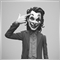29938

Pierre Rougier:

Hello,

How to know the number of the week?

MqlDateTime gives a lot of info, but I did not find the number of the week.

```int StdWeek(){
int iDay  = (DayOfWeek() + 6 ) % 7 + 1;        // convert day to standard index (1=Mon,...,7=Sun)
int iWeek = (DayOfYear() - iDay + 10 ) / 7;    // calculate standard week number
return iWeek;
}```5418

Mohamad Zulhairi Baba:

OK, so looking at this as MQL5 code:

```int StdWeek(MqlDateTime &dt){
int iDay  = (dt.day_of_week + 6 ) % 7 + 1;        // convert day to standard index (1=Mon,...,7=Sun)
int iWeek = (dt.day_of_year - iDay + 10 ) / 7;    // calculate standard week number
return iWeek;
}```

For 2016, it gives:

Jan 1: 0
Jan 2: 0
Jan 3: 0
Jan 4: 1

Assuming the first week starts at 0 (instead of 1), we're OK until Jan. 3, 2016. In the US, Sundays start the week. So this formula may be correct if you want Sundays to be on the previous week and the first week starts at 0 instead of 1. That's as much testing as I have done. Caveat emptor.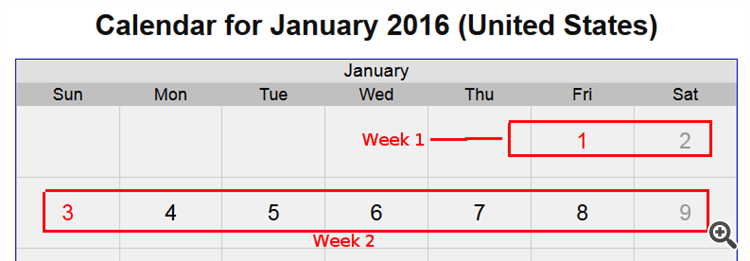802

Thanks to all.

I made a script, maybe can help someone.

```//+------------------------------------------------------------------+
//|                                                  WeekNumber2.mq5 |
//|                                   Copyright 2018, Pierre Rougier |
//|                                        http://apprendre-mql5.com |
//+------------------------------------------------------------------+
#property copyright "Copyright 2018, Pierre Rougier"
#property link      "http://apprendre-mql5.com"
#property version   "1.00"
//+------------------------------------------------------------------+
//|                                                                  |
//+------------------------------------------------------------------+
datetime YearStartTime(datetime aTime)
{
MqlDateTime stm;
TimeToStruct(aTime,stm);
stm.day=1;
stm.mon=1;
stm.hour=0;
stm.min=0;
stm.sec=0;
return(StructToTime(stm));
}
//+------------------------------------------------------------------+
//|                                                                  |
//+------------------------------------------------------------------+
long WeekNumFromDate(datetime aTime,datetime aStartTime,bool aStartsOnMonday=false)
{
long Time,StartTime,Corrector;
MqlDateTime stm;
Time=aTime;
StartTime=aStartTime;
//--- determine the beginning of the reference epoch
StartTime=(StartTime/86400)*86400;
//--- determine the time that elapsed since the beginning of the reference epoch
Time-=StartTime;
//--- determine the day of the week of the beginning of the reference epoch
TimeToStruct(StartTime,stm);
//--- if the week starts on Monday, numbers of days of the week are decreased by 1,
//    and the day with number 0  becomes a day with number 6
if(aStartsOnMonday)
{
if(stm.day_of_week==0)
{
stm.day_of_week=6;
}
else
{
stm.day_of_week--;
}
}
//--- calculate the value of the time corrector
Corrector=86400*stm.day_of_week;
//--- time correction
Time+=Corrector;
//--- calculate and return the number of the week
return(Time/604800);
}
//+------------------------------------------------------------------+
//|                                                                  |
//+------------------------------------------------------------------+
long WeekNumYear(datetime aTime,bool aStartsOnMonday=false)
{
return(WeekNumFromDate(aTime,YearStartTime(aTime),aStartsOnMonday));
}
//+------------------------------------------------------------------+
//|                                                                  |
//+------------------------------------------------------------------+
int StdWeek(MqlDateTime &dt)
{
int iDay  = (dt.day_of_week + 6 ) % 7 + 1;        // convert day to standard index (1=Mon,...,7=Sun)
int iWeek = (dt.day_of_year - iDay + 10 ) / 7;    // calculate standard week number
return iWeek;
}
//+------------------------------------------------------------------+
//| Script program start function                                    |
//+------------------------------------------------------------------+
void OnStart()
{
//---

//***************  WeekNumYear ******************
//***********************************************

datetime date_2019_12_31=D'2019.12.31 12:30';
datetime date_2020_01_01=D'2020.01.01 12:30';
datetime date_2020_01_05=D'2020.01.05 12:30';
datetime date_2020_01_06=D'2020.01.06 12:30';
datetime date_2020_01_12=D'2020.01.12 12:30';
datetime date_2020_01_13=D'2020.01.13 12:30';
datetime date_2020_01_19=D'2020.01.19 12:30';

printf("WeekNumYear(date_2019_12_31,true) :%02d",WeekNumYear(date_2019_12_31,true));
printf("WeekNumYear(date_2020_01_01,true) :%02d",WeekNumYear(date_2020_01_01,true));
printf("WeekNumYear(date_2020_01_05,true) :%02d",WeekNumYear(date_2020_01_05,true));
printf("WeekNumYear(date_2020_01_06,true) :%02d",WeekNumYear(date_2020_01_06,true));
printf("WeekNumYear(date_2020_01_12,true) :%02d",WeekNumYear(date_2020_01_12,true));
printf("WeekNumYear(date_2020_01_13,true) :%02d",WeekNumYear(date_2020_01_13,true));
printf("WeekNumYear(date_2020_01_19,true) :%02d",WeekNumYear(date_2020_01_19,true));
Print("");

printf("WeekNumYear(date_2019_12_31,false) :%02d",WeekNumYear(date_2019_12_31,false));
printf("WeekNumYear(date_2020_01_01,false) :%02d",WeekNumYear(date_2020_01_01,false));
printf("WeekNumYear(date_2020_01_05,false) :%02d",WeekNumYear(date_2020_01_05,false));
printf("WeekNumYear(date_2020_01_06,false) :%02d",WeekNumYear(date_2020_01_06,false));
printf("WeekNumYear(date_2020_01_12,false) :%02d",WeekNumYear(date_2020_01_12,false));
printf("WeekNumYear(date_2020_01_13,false) :%02d",WeekNumYear(date_2020_01_13,false));
printf("WeekNumYear(date_2020_01_19,false) :%02d",WeekNumYear(date_2020_01_19,false));
Print("");

//*****************  StdWeek ********************
//***********************************************

MqlDateTime MDT_2019_12_31,MDT_2020_01_01,MDT_2020_01_05,MDT_2020_01_06,
MDT_2020_01_12,MDT_2020_01_13,MDT_2020_01_19;

TimeToStruct(date_2019_12_31,MDT_2019_12_31);
TimeToStruct(date_2020_01_01,MDT_2020_01_01);
TimeToStruct(date_2020_01_05,MDT_2020_01_05);
TimeToStruct(date_2020_01_06,MDT_2020_01_06);
TimeToStruct(date_2020_01_12,MDT_2020_01_12);
TimeToStruct(date_2020_01_13,MDT_2020_01_13);
TimeToStruct(date_2020_01_19,MDT_2020_01_19);

printf("StdWeek(MDT_2019_12_31) :%02d",StdWeek(MDT_2019_12_31));
printf("StdWeek(MDT_2020_01_01) :%02d",StdWeek(MDT_2020_01_01));
printf("StdWeek(MDT_2020_01_05) :%02d",StdWeek(MDT_2020_01_05));
printf("StdWeek(MDT_2020_01_06) :%02d",StdWeek(MDT_2020_01_06));
printf("StdWeek(MDT_2020_01_12) :%02d",StdWeek(MDT_2020_01_12));
printf("StdWeek(MDT_2020_01_13) :%02d",StdWeek(MDT_2020_01_13));
printf("StdWeek(MDT_2020_01_19) :%02d",StdWeek(MDT_2020_01_19));

}
//+------------------------------------------------------------------+
```